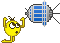# resolving fraction when X is added in the numerator *and* denominator

#### jedidia

##### shoemaker without legs
This is a very basic question about algebra, but it's been... quite a while, and my education sucks anyways. Googling didn't really yield anything, I'm probably not looking for the right terms.

So to illustrate, let's say I have a fraction with X on both sides, and I have the ratio, for example like so:

(5 + x) / (2 + x) = 1.75

Obviously, x = 2 in this case. I've made the example up to demonstrate, after all.
But I can't for the life of me figure out how to solve this for x. I just don't remember the rules for additions in fractions and I can't seem to find them without reading a textbook front to back...

Last edited:
•Notebook

#### GLS

##### Well-known member
Orbiter Contributor
That is solvable by shuffling terms around*, but if I remember correctly, this is the more general solution: https://en.wikipedia.org/wiki/Polynomial_long_division

*) pass the 2+x to the right side and multiply it by the 1.75 there, then pass the 5, then divide everything by x (the "trick", so you end up with only 1 x in the expression), and then it should be easy.

••jedidia and Notebook

#### jedidia

##### shoemaker without legs
Right, got it, thanks a whole bunch! The result of the entire thing, in case anybody's interested or can use it:

The maximum payload that a spacecraft can carry for a given mission-DV:
dv: delta-velocity (m/s), given
ev: exhaust velocity (m/s), given
M0: Full mass with propellant (but without payload, obviously) (kg), given
M1: Dry mass (kg), given

First simplify by reducing dv and ev to mass ratio, because otherwise I get lost in the terms:
Mr = exp(dv/ev)

starting point:
Mr = (M0 + x) / (M1 + x)
mutiply by M1 + x:
Mrx + MrM1 = M0 + x
subtract M0:
Mrx + MrM1 - M0 = x
divide by x:
Mrx / x + (MrM1 - M0) / x = 1
Which is equivalent to:
(Mr + M1 - M0) / x + Mr = 1
subtract Mr:
(Mr + M1 - M0) / x = 1 -Mr
Get x out of there:
Mr + M1 - M0 = x(1-Mr)
Finally, divide by 1-Mr, and I'm done:
(Mr + M1 - M0) / (1 - Mr) = x

Ran it with some numbers, and seems to check out.
Thanks again for the help!

•Notebook

#### Boxx

Donator
Warning, if you make it shorter, you've got this:

starting point:
Mr = (M0 + x) / (M1 + x)
mutiply by M1 + x:
Mrx + MrM1 = M0 + x
subtract M0:
Mrx + MrM1 - M0 = x
from here, pass x to the left and MrM1-M0 to the right, changing the signs:
Mrx - x = M0-MrM1
then (Mr-1)x = M0-MrM1
then x = (M0-MrM1)/(Mr-1)

(there was a mistake here)
Mrx / x + (MrM1 - M0) / x = 1
Which is NOT equivalent to:
(Mr + M1 - M0) / x + Mr = 1

Last edited:
•jedidia and Notebook

#### jedidia

##### shoemaker without legs
(there was a mistake here)

Whoops. Now I'm wondering why my equation provides results that seem to work. Guess math is like code sometimes...•Matias Saibene